### TEST Statement

<label:> TEST equation1 <, equation2, …> </ option> ;

The TEST statement tests linear hypotheses about the regression coefficients. The Wald test is used to perform a joint test of the null hypotheses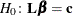specified in a single TEST statement, whereis the vector of intercept and slope parameters. When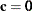you should specify a CONTRAST statement instead.

Each equation specifies a linear hypothesis (a row of thematrix and the corresponding element of thevector). Multiple equations are separated by commas. The label, which must be a valid SAS name, is used to identify the resulting output and should always be included. You can submit multiple TEST statements.

The form of an equation is as follows:

term<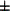term> <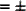term <term>>

where term is a parameter of the model, or a constant, or a constant times a parameter. Intercept and CLASS variable parameter names should be specified as described in the section Parameter Names in the OUTEST= Data Set. Note for generalized logit models that this enables you to construct tests of parameters from specific logits. When no equal sign appears, the expression is set to 0. The following statements illustrate possible uses of the TEST statement:

proc logistic;
model y= a1 a2 a3 a4;
test1: test intercept + .5 * a2 = 0;
test2: test intercept + .5 * a2;
test3: test a1=a2=a3;
test4: test a1=a2, a2=a3;
run;


Note that the first and second TEST statements are equivalent, as are the third and fourth TEST statements.

You can specify the following option in the TEST statement after a slash(/):

PRINT

displays intermediate calculations in the testing of the null hypothesis. This includes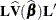bordered by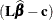and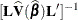bordered by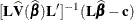, where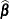is the maximum likelihood estimator ofand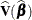is the estimated covariance matrix of.